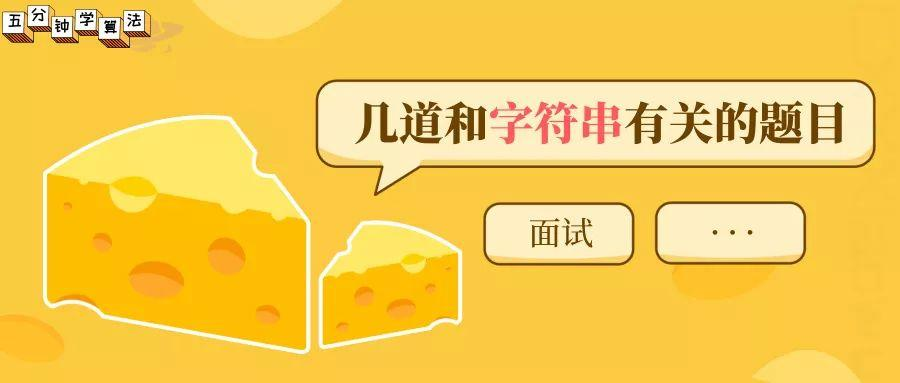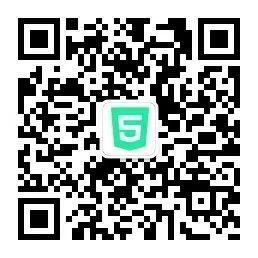String 作为最常见的编程语言类型之一，在算法面试中出现的频率极高。

## 1. 验证回文串

### 题目描述

输入: "A man, a plan, a canal: Panama"输出: true

输入: "race a car"输出: false

### 代码实现

class Solution {    public boolean isPalindrome(String s) {        if(s.length() == 0)             return true;        int l = 0, r = s.length() - 1;        while(l < r){            //确定指定的字符是否为字母或数字            if(!Character.isLetterOrDigit(s.charAt(l))){                l++;            }else if(!Character.isLetterOrDigit(s.charAt(r))){                r--;            }else{                if(Character.toLowerCase(s.charAt(l)) != Character.toLowerCase(s.charAt(r)))                    return false;                l++;                r--;            }         }        return true;    }}

## 2. 分割回文串

### 题目描述

输入: "aab"输出:[  ["aa","b"],  ["a","a","b"]]

### 题目解析

1. 分割为 a + ac

2. 分割为 a + a + c，分割后，得到一组结果，再回溯到  a + ac

3. a + ac 中 ac 不是回文串，继续回溯，回溯到 aac

4. 分割为稍长的回文串，分割为 aa + c 分割完成得到一组结果，再回溯到 aac

5. aac 不是回文串，搜索结束

### 代码实现

class Solution {    List<List<String>> res = new ArrayList<>();    public List<List<String>> partition(String s) {        if(s==null||s.length()==0)            return res;        dfs(s,new ArrayList<String>(),0);        return res;    }    public void dfs(String s,List<String> remain,int left){        if(left==s.length()){  //判断终止条件            res.add(new ArrayList<String>(remain));  //添加到结果中            return;        }        for(int right=left;right<s.length();right++){  //从left开始，依次判断left->right是不是回文串            if(isPalindroom(s,left,right)){  //判断是否是回文串                remain.add(s.substring(left,right+1));   //添加到当前回文串到list中                dfs(s,remain,right+1);  //从right+1开始继续递归，寻找回文串                remain.remove(remain.size()-1);  //回溯，从而寻找更长的回文串            }        }    }    /**    * 判断是否是回文串    */    public boolean isPalindroom(String s,int left,int right){        while(left<right&&s.charAt(left)==s.charAt(right)){            left++;            right--;        }        return left>=right;    }}

## 3. 单词拆分

### 题目描述

• 拆分时可以重复使用字典中的单词。

• 你可以假设字典中没有重复的单词。

### 代码实现

class Solution {    public boolean wordBreak(String s, List<String> wordDict) {        int n = s.length();        int max_length=0;        for(String temp:wordDict){            max_length = temp.length() > max_length ? temp.length() : max_length;        }        // memo[i] 表示 s 中以 i - 1 结尾的字符串是否可被 wordDict 拆分        boolean[] memo = new boolean[n + 1];        memo = true;        for (int i = 1; i <= n; i++) {            for (int j = i-1; j >= 0 && max_length >= i - j; j--) {                if (memo[j] && wordDict.contains(s.substring(j, i))) {                    memo[i] = true;                    break;                }            }        }        return memo[n];    }}

## 4. 反转字符串

### 题目描述

输入：["h","e","l","l","o"]输出：["o","l","l","e","h"]

输入：["H","a","n","n","a","h"]输出：["h","a","n","n","a","H"]

### 代码实现

class Solution {public:    string reverseString(string s) {        int i = 0, j = s.size() - 1;        while (i < j){            swap(s[i],s[j]);            i++;            j--;        }        return s;    }};

## 5. 把字符串转换成整数

### 题目解析

• 指针是否为空指针以及字符串是否为空字符串；

• 字符串对于正负号的处理；

• 输入值是否为合法值，即小于等于’9’，大于等于’0’；

• int为32位，需要判断是否溢出；

• 使用错误标志，区分合法值0和非法值0。

### 代码实现

public class Solution {    public int StrToInt(String str) {    if (str == null || str.length() == 0)        return 0;    boolean isNegative = str.charAt(0) == '-';    int ret = 0;    for (int i = 0; i < str.length(); i++) {        char c = str.charAt(i);        if (i == 0 && (c == '+' || c == '-'))  /* 符号判定 */            continue;        if (c < '0' || c > '9')                /* 非法输入 */            return 0;        ret = ret * 10 + (c - '0');    }    return isNegative ? -ret : ret;  }}